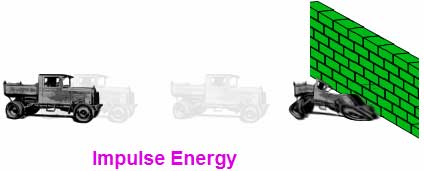# Impulse Formula

## Impulse

A car is travelling at full speed. It crashes into the barrier, because of the negligence of the driver.Here the huge force is applied on the wall by the car in a very little time interval which we term as an impulse.

Impulse is the big force acting for a very small interval of time. It is represented by J⃗ J→.

Impulse Formula is articulated as

J=F×t

Where,

• Force applied is given as F
• Time interval throughout which force is applied is given as t.

Impulse can also be articulated as the rate of change of momentum.

J=m×v

Where,

• Mass of the body is given as m
• The velocity with which the body is moving is given as v.

Velocity is articulated as

v=vf – vi

Where,

• Initial Velocity is given as vi
• Final Velocity is given as vf .

Therefore, the Impulsive force is articulated as

$$\begin{array}{l}f=m\frac{v_{f}-v_{i}}{t}\end{array}$$

Impulse is articulated in Kgms-1 and Impulsive force is articulated in Newton(N).

### Impulse-momentum formula

The Impulse-momentum formula is obtained from the impulse-momentum theorem which states that change in momentum of an object is equal to impulse applied on the object. The formula is given as follows:

 Impulse-momentum formula J=Δp When the mass is constant FΔt=mΔv When the mass is varying Fdt=mdv+vdm

As the SI unit of impulse and momentum are equal, it is given as Ns=kg.m.s-1

### Solved Examples

Below are some problems on impulse:

Example 1: A batsman knocks back a ball straight in the direction towards the bowler without altering its initial speed of 12 m/s. If the mass of the ball is 0.15kg, calculate the impulse imparted to the ball?

Given:
vi (Initial Velocity) = 12 m/s,

vf (Final Velocity) = -12m/s,

m (mass) = 0.15kg,

J (Impulse) = ?

Impulse is articulated as

J = mvf – mvi

= m(vf – vi)

= 0.15 Kg (-12 -12) m/s

J= -3.6N.

Example 2: A golfer hits a ball of mass 45g at a speed of 40m/s. The golf club is in contact with the ball for 3 s. Compute the average force applied by the club on the ball?

m (Mass)= 0.045 kg,

vi (Initial Velocity) = 0,

vf (Final Velocity) = 40m/s,

Impulse force F = ma =

$$\begin{array}{l}m\frac{v_{f}-v_{i}}{t}\end{array}$$
$$\begin{array}{l}=0.045 \times \frac{40}{0.003}\end{array}$$

F= 600N